# AN INTRODUCTION TO ORGANIC CHEMISTRY A guide for

• Slides: 44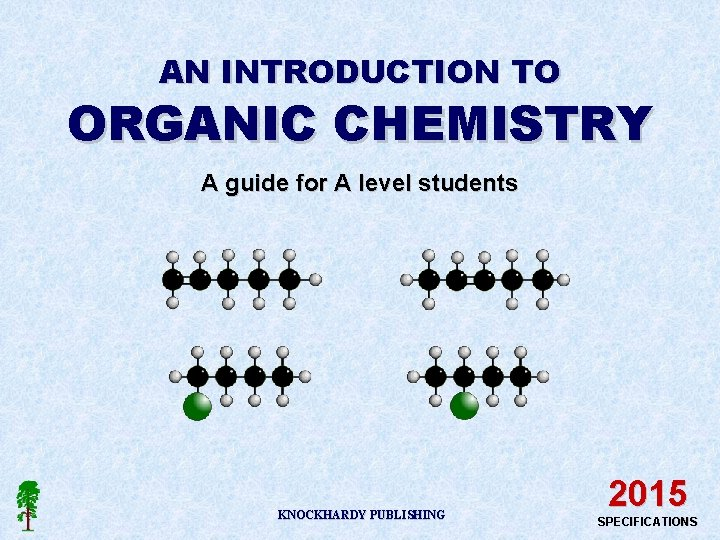AN INTRODUCTION TO ORGANIC CHEMISTRY A guide for A level students KNOCKHARDY PUBLISHING 2015 SPECIFICATIONS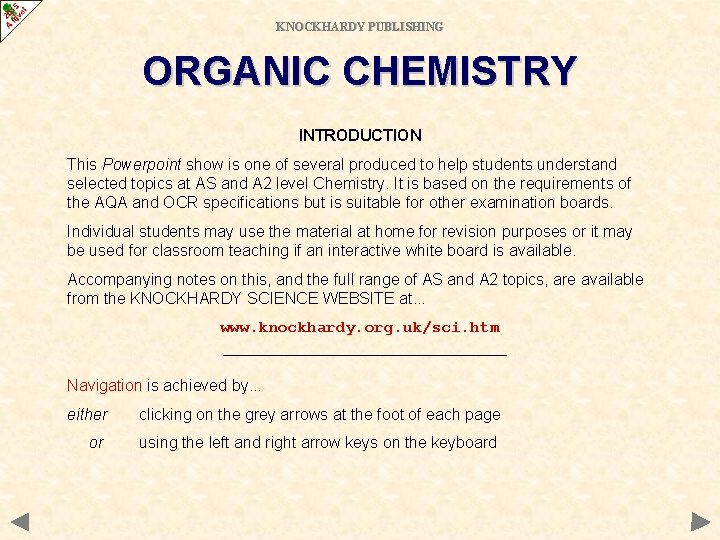KNOCKHARDY PUBLISHING ORGANIC CHEMISTRY INTRODUCTION This Powerpoint show is one of several produced to help students understand selected topics at AS and A 2 level Chemistry. It is based on the requirements of the AQA and OCR specifications but is suitable for other examination boards. Individual students may use the material at home for revision purposes or it may be used for classroom teaching if an interactive white board is available. Accompanying notes on this, and the full range of AS and A 2 topics, are available from the KNOCKHARDY SCIENCE WEBSITE at. . . www. knockhardy. org. uk/sci. htm Navigation is achieved by. . . either clicking on the grey arrows at the foot of each page or using the left and right arrow keys on the keyboard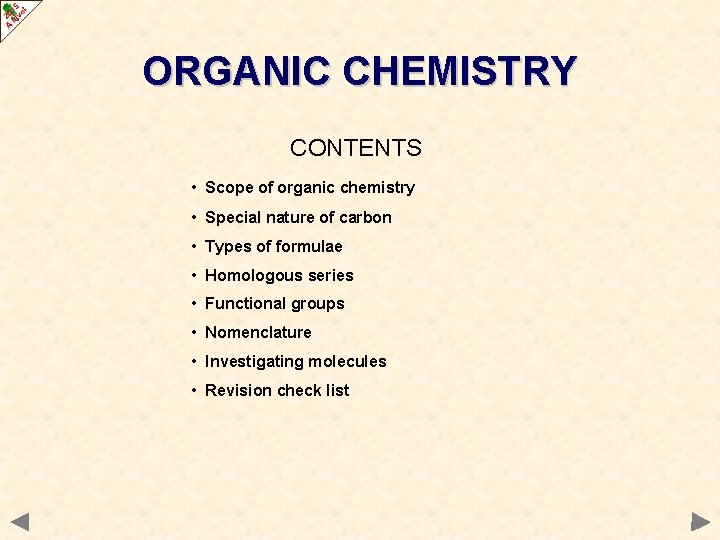ORGANIC CHEMISTRY CONTENTS • Scope of organic chemistry • Special nature of carbon • Types of formulae • Homologous series • Functional groups • Nomenclature • Investigating molecules • Revision check list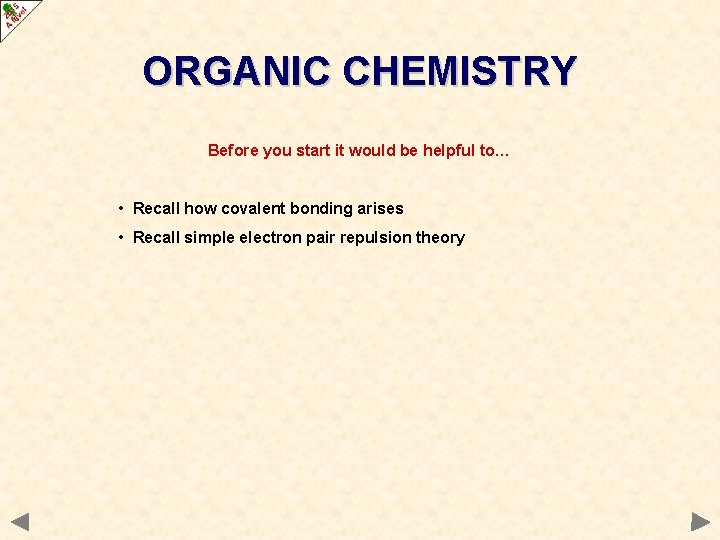ORGANIC CHEMISTRY Before you start it would be helpful to… • Recall how covalent bonding arises • Recall simple electron pair repulsion theory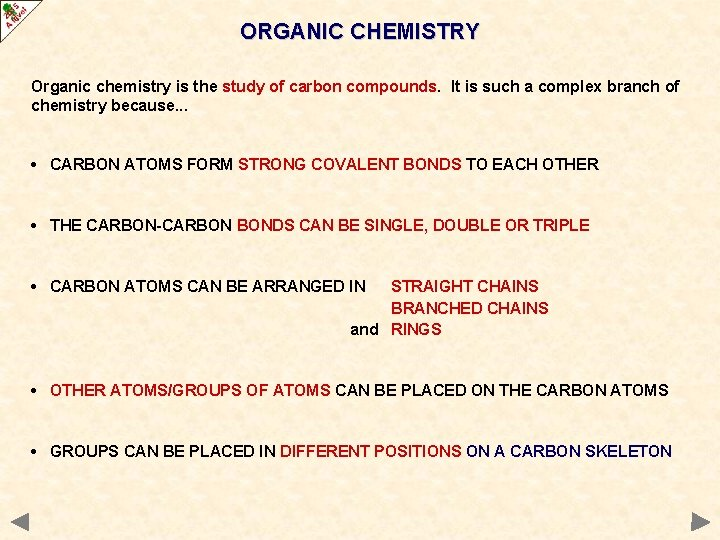ORGANIC CHEMISTRY Organic chemistry is the study of carbon compounds. It is such a complex branch of chemistry because. . . • CARBON ATOMS FORM STRONG COVALENT BONDS TO EACH OTHER • THE CARBON-CARBON BONDS CAN BE SINGLE, DOUBLE OR TRIPLE • CARBON ATOMS CAN BE ARRANGED IN STRAIGHT CHAINS BRANCHED CHAINS and RINGS • OTHER ATOMS/GROUPS OF ATOMS CAN BE PLACED ON THE CARBON ATOMS • GROUPS CAN BE PLACED IN DIFFERENT POSITIONS ON A CARBON SKELETON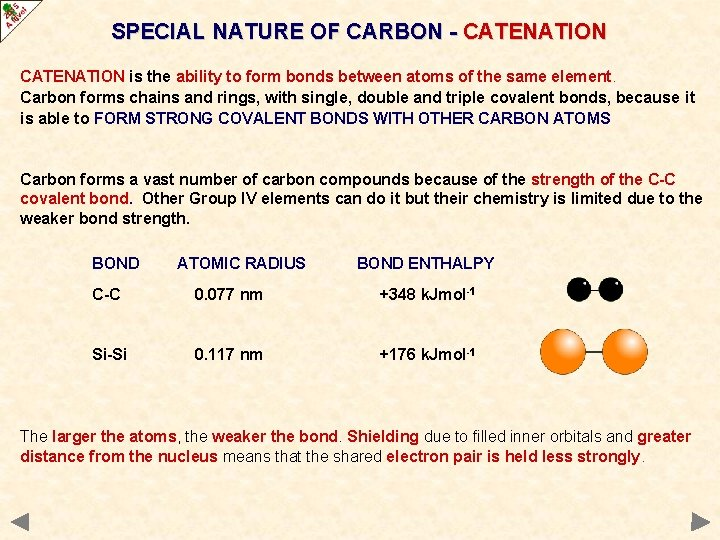SPECIAL NATURE OF CARBON - CATENATION is the ability to form bonds between atoms of the same element. Carbon forms chains and rings, with single, double and triple covalent bonds, because it is able to FORM STRONG COVALENT BONDS WITH OTHER CARBON ATOMS Carbon forms a vast number of carbon compounds because of the strength of the C-C covalent bond. Other Group IV elements can do it but their chemistry is limited due to the weaker bond strength. BOND ATOMIC RADIUS BOND ENTHALPY C-C 0. 077 nm +348 k. Jmol-1 Si-Si 0. 117 nm +176 k. Jmol-1 The larger the atoms, the weaker the bond. Shielding due to filled inner orbitals and greater distance from the nucleus means that the shared electron pair is held less strongly.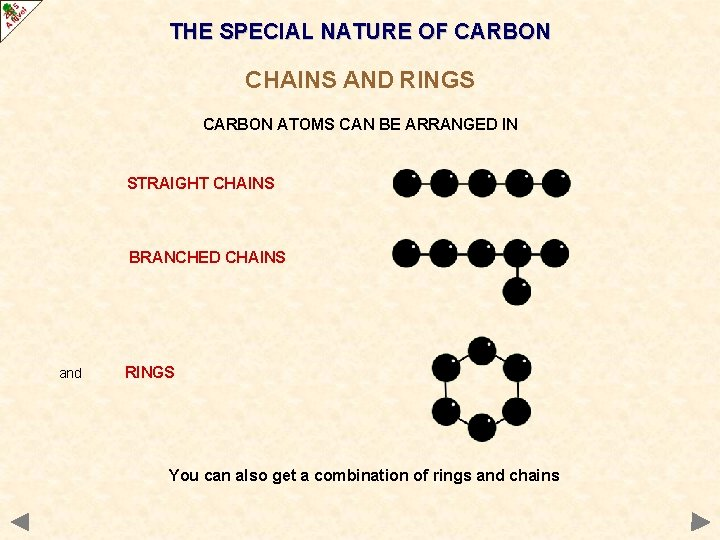THE SPECIAL NATURE OF CARBON CHAINS AND RINGS CARBON ATOMS CAN BE ARRANGED IN STRAIGHT CHAINS BRANCHED CHAINS and RINGS You can also get a combination of rings and chains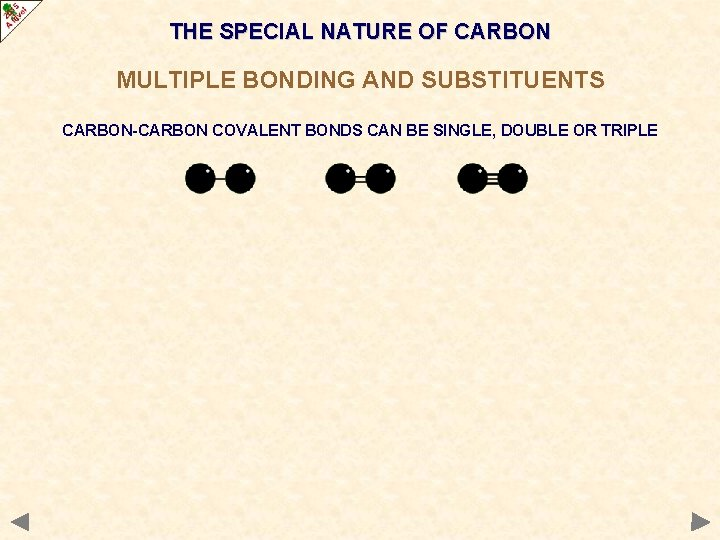THE SPECIAL NATURE OF CARBON MULTIPLE BONDING AND SUBSTITUENTS CARBON-CARBON COVALENT BONDS CAN BE SINGLE, DOUBLE OR TRIPLETHE SPECIAL NATURE OF CARBON MULTIPLE BONDING AND SUBSTITUENTS CARBON-CARBON COVALENT BONDS CAN BE SINGLE, DOUBLE OR TRIPLE DIFFERENT ATOMS / GROUPS OF ATOMS CAN BE PLACED ON THE CARBONS The basic atom is HYDROGEN but groups containing OXYGEN, NITROGEN, HALOGENS and SULPHUR are very common. CARBON SKELETON FUNCTIONAL GROUP The chemistry of an organic compound is determined by its FUNCTIONAL GROUPTHE SPECIAL NATURE OF CARBON MULTIPLE BONDING AND SUBSTITUENTS ATOMS/GROUPS CAN BE PLACED IN DIFFERENT POSITIONS ON A CARBON SKELETON THE C=C DOUBLE BOND IS IN A DIFFERENT POSITION PENT-1 -ENE PENT-2 -ENE THE CHLORINE ATOM IS IN A DIFFERENT POSITION 1 -CHLOROBUTANE 2 -CHLOROBUTANE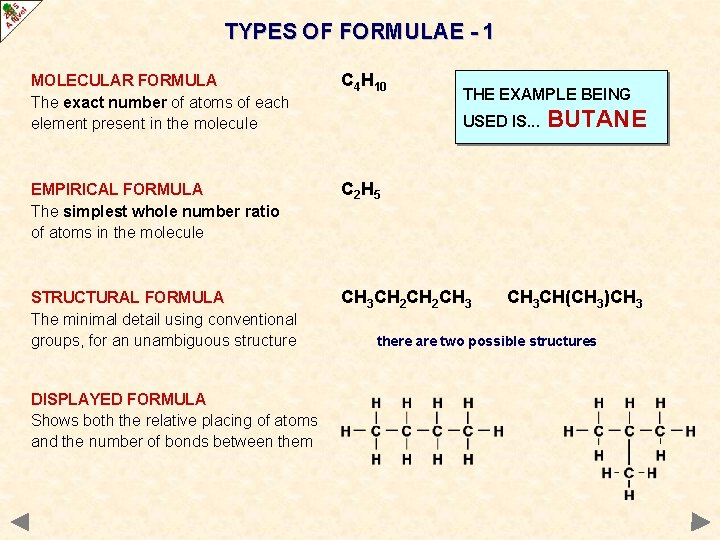TYPES OF FORMULAE - 1 MOLECULAR FORMULA The exact number of atoms of each element present in the molecule C 4 H 10 EMPIRICAL FORMULA The simplest whole number ratio of atoms in the molecule C 2 H 5 STRUCTURAL FORMULA The minimal detail using conventional groups, for an unambiguous structure CH 3 CH 2 CH 3 DISPLAYED FORMULA Shows both the relative placing of atoms and the number of bonds between them THE EXAMPLE BEING USED IS. . . BUTANE CH 3 CH(CH 3)CH 3 there are two possible structuresTYPES OF FORMULAE - 2 SKELETAL FORMULA A skeletal formula is used to show a simplified organic formula by removing hydrogen atoms from alkyl chains, leaving just a carbon skeleton and associated functional groups for CYCLOHEXANE THALIDOMIDE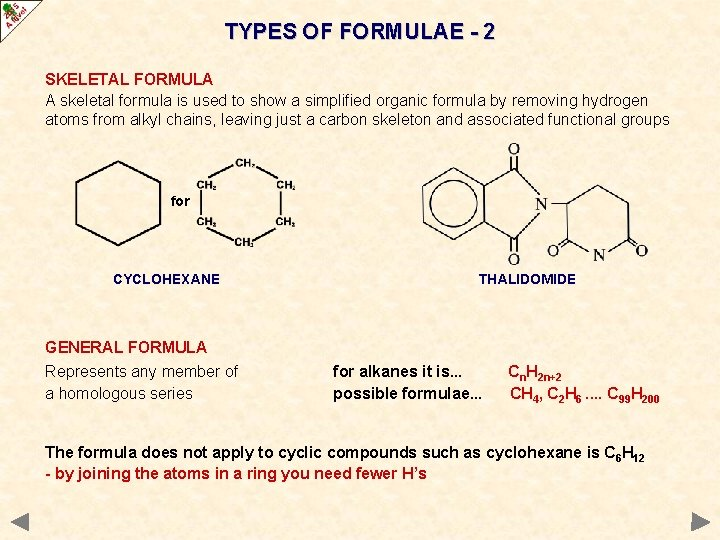TYPES OF FORMULAE - 2 SKELETAL FORMULA A skeletal formula is used to show a simplified organic formula by removing hydrogen atoms from alkyl chains, leaving just a carbon skeleton and associated functional groups for CYCLOHEXANE THALIDOMIDE GENERAL FORMULA Represents any member of a homologous series for alkanes it is. . . possible formulae. . . Cn. H 2 n+2 CH 4, C 2 H 6. . C 99 H 200 The formula does not apply to cyclic compounds such as cyclohexane is C 6 H 12 - by joining the atoms in a ring you need fewer H’sHOMOLOGOUS SERIES A series of compounds of similar structure in which each member differs from the next by a common repeating unit, CH 2. Series members are called homologues and. . . • all share the same general formula. • formula of a homologue differs from its neighbour by CH 2. (e. g. CH 4, C 2 H 6, . . . etc ) • contain the same functional group • have similar chemical properties. • show a gradual change in physical properties as molar mass increases. • can usually be prepared by similar methods. ALCOHOLS - FIRST THREE MEMBERS OF THE SERIES CH 3 OH METHANOL C 2 H 5 OH ETHANOL C 3 H 7 OH PROPAN-1 -OLFUNCTIONAL GROUPS Organic chemistry is a vast subject so it is easier to split it into small sections for study. This is done by studying compounds which behave in a similar way because they have a particular atom, or group of atoms, FUNCTIONAL GROUP, in their structure. Functional groups can consist of one atom, a group of atoms or multiple bonds between carbon atoms. Each functional group has its own distinctive properties which means that the properties of a compound are governed by the functional group(s) in it. H H H C C C NH 2 OH H H Carbon skeleton H C C C Functional Group = AMINE Carbon skeleton Functional Group = ALCOHOL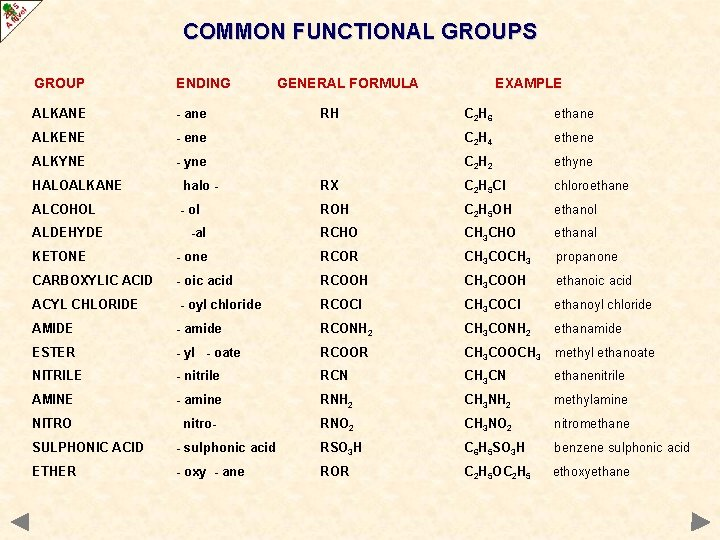COMMON FUNCTIONAL GROUPS GROUP ENDING ALKANE - ane ALKENE ALKYNE GENERAL FORMULA RH EXAMPLE C 2 H 6 ethane - ene C 2 H 4 ethene - yne C 2 H 2 ethyne HALOALKANE halo - RX C 2 H 5 Cl chloroethane ALCOHOL - ol ROH C 2 H 5 OH ethanol -al RCHO CH 3 CHO ethanal KETONE - one RCOR CH 3 COCH 3 propanone CARBOXYLIC ACID - oic acid RCOOH CH 3 COOH ethanoic acid ACYL CHLORIDE - oyl chloride RCOCl CH 3 COCl ethanoyl chloride AMIDE - amide RCONH 2 CH 3 CONH 2 ethanamide ESTER - yl - oate RCOOR CH 3 COOCH 3 methyl ethanoate NITRILE - nitrile RCN CH 3 CN ethanenitrile AMINE - amine RNH 2 CH 3 NH 2 methylamine NITRO nitro- RNO 2 CH 3 NO 2 nitromethane ALDEHYDE SULPHONIC ACID - sulphonic acid RSO 3 H C 6 H 5 SO 3 H benzene sulphonic acid ETHER - oxy - ane ROR C 2 H 5 OC 2 H 5 ethoxyethane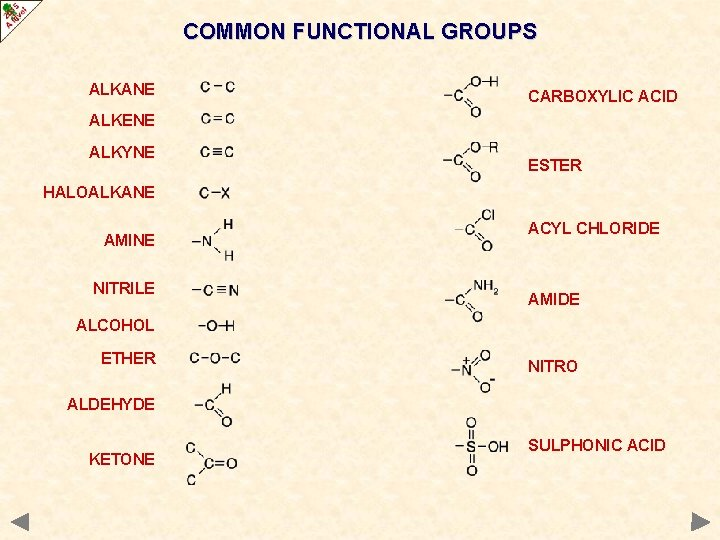COMMON FUNCTIONAL GROUPS ALKANE CARBOXYLIC ACID ALKENE ALKYNE ESTER HALOALKANE AMINE NITRILE ACYL CHLORIDE AMIDE ALCOHOL ETHER NITRO ALDEHYDE KETONE SULPHONIC ACIDHOW MANY STRUCTURES? Draw legitimate structures for each molecular formula and classify each one according to the functional group present. Not all the structures represent stable compounds. carbon atoms have oxygen atoms nitrogen atoms hydrogen halogen atoms 4 covalent bonds surrounding them 2 3 1 1 C 2 H 6 ONE C 3 H 7 Br TWO C 4 H 8 FIVE - 3 with C=C and 2 ring compounds with all C-C’s C 2 H 6 O TWO - 1 with C-O-C and 1 with C-O-H C 3 H 6 O SIX - 2 with C=O, 2 with C=C and 2 with rings C 2 H 7 N TWO C 2 H 4 O 2 SEVERAL - Only 2 are stable C 2 H 3 N TWOHOW MANY STRUCTURES? Draw legitimate structures for each molecular formula and classify each one according to the functional group present. Not all the structures represent stable compounds. carbon atoms have oxygen atoms nitrogen atoms hydrogen halogen atoms 4 covalent bonds surrounding them 2 3 1 1 C 2 H 6 ONE C 3 H 7 Br TWO C 4 H 8 FIVE - 3 with C=C and 2 ring compounds with all C-C’s C 2 H 6 O TWO - 1 with C-O-C and 1 with C-O-H C 3 H 6 O SIX - 2 with C=O, 2 with C=C and 2 with rings C 2 H 7 N TWO C 2 H 4 O 2 SEVERAL - Only 2 are stable C 2 H 3 N TWO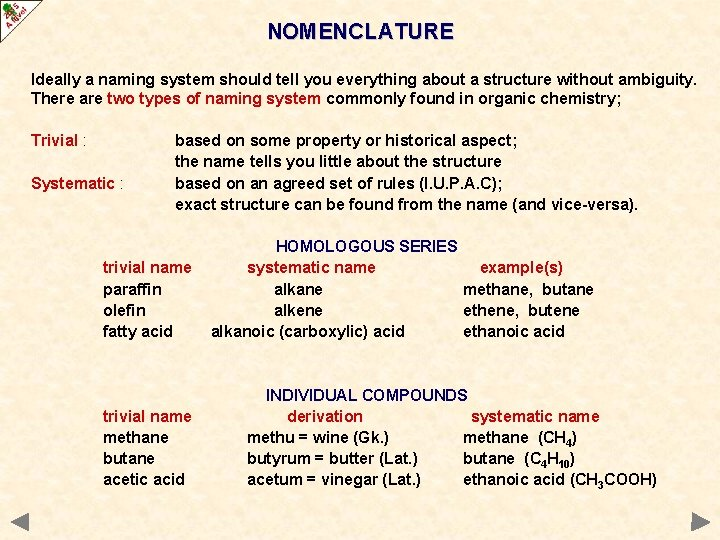NOMENCLATURE Ideally a naming system should tell you everything about a structure without ambiguity. There are two types of naming system commonly found in organic chemistry; Trivial : Systematic : based on some property or historical aspect; the name tells you little about the structure based on an agreed set of rules (I. U. P. A. C); exact structure can be found from the name (and vice-versa). trivial name paraffin olefin fatty acid trivial name methane butane acetic acid HOMOLOGOUS SERIES systematic name example(s) alkane methane, butane alkene ethene, butene alkanoic (carboxylic) acid ethanoic acid INDIVIDUAL COMPOUNDS derivation systematic name methu = wine (Gk. ) methane (CH 4) butyrum = butter (Lat. ) butane (C 4 H 10) acetum = vinegar (Lat. ) ethanoic acid (CH 3 COOH)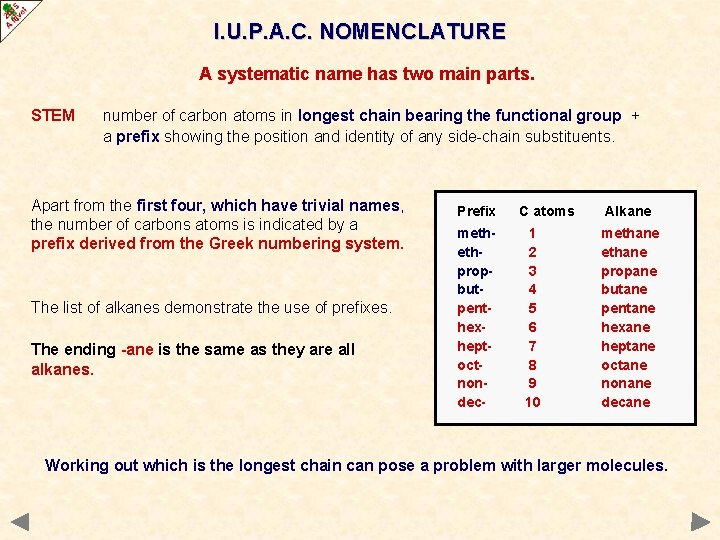I. U. P. A. C. NOMENCLATURE A systematic name has two main parts. STEM number of carbon atoms in longest chain bearing the functional group + a prefix showing the position and identity of any side-chain substituents. Apart from the first four, which have trivial names, the number of carbons atoms is indicated by a prefix derived from the Greek numbering system. The list of alkanes demonstrate the use of prefixes. The ending -ane is the same as they are all alkanes. Prefix methethpropbutpenthexheptoctnondec- C atoms 1 2 3 4 5 6 7 8 9 10 Alkane methane propane butane pentane hexane heptane octane nonane decane Working out which is the longest chain can pose a problem with larger molecules.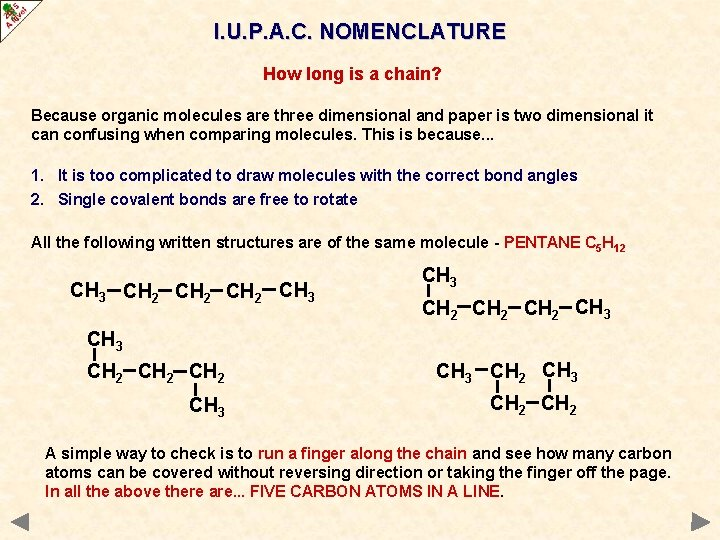I. U. P. A. C. NOMENCLATURE How long is a chain? Because organic molecules are three dimensional and paper is two dimensional it can confusing when comparing molecules. This is because. . . 1. It is too complicated to draw molecules with the correct bond angles 2. Single covalent bonds are free to rotate All the following written structures are of the same molecule - PENTANE C 5 H 12 CH 3 CH 2 CH 2 CH 3 CH 3 CH 2 A simple way to check is to run a finger along the chain and see how many carbon atoms can be covered without reversing direction or taking the finger off the page. In all the above there are. . . FIVE CARBON ATOMS IN A LINE.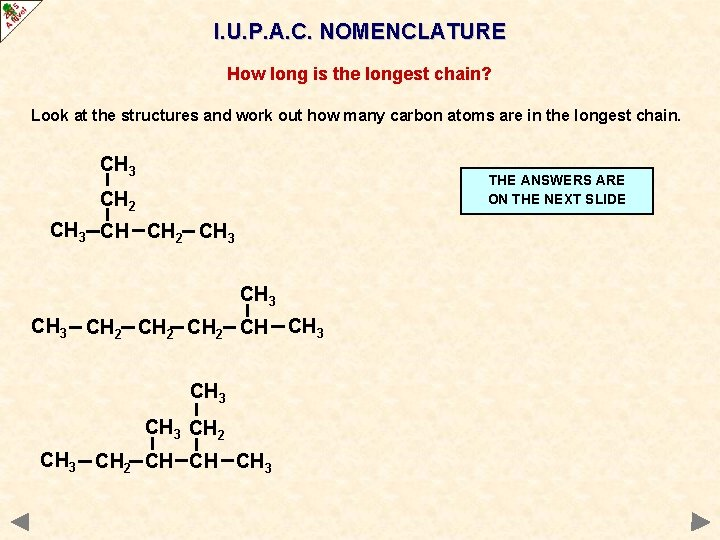I. U. P. A. C. NOMENCLATURE How long is the longest chain? Look at the structures and work out how many carbon atoms are in the longest chain. CH 3 THE ANSWERS ARE ON THE NEXT SLIDE CH 2 CH 3 CH 2 CH CH 3 CH 2 CH CH CH 3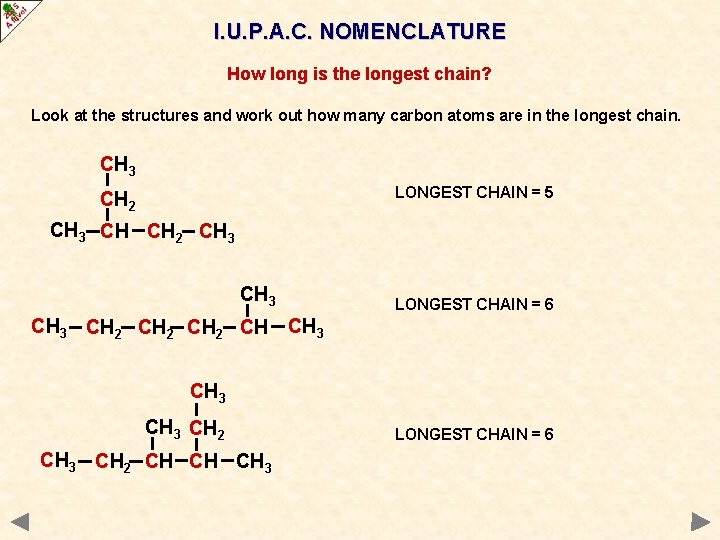I. U. P. A. C. NOMENCLATURE How long is the longest chain? Look at the structures and work out how many carbon atoms are in the longest chain. CH 3 LONGEST CHAIN = 5 CH 2 CH 3 LONGEST CHAIN = 6 CH 3 CH 2 CH CH 3 CH 2 CH CH CH 3 LONGEST CHAIN = 6I. U. P. A. C. NOMENCLATURE A systematic name has two main parts. SUFFIX An ending that tells you which functional group is present See if any functional groups are present. Add relevant ending to the basic stem. In many cases the position of the functional group must be given to avoid any ambiguity 1 -CHLOROBUTANE SUBSTITUENTS Functional group Suffix ALKANE ALKENE ALKYNE ALCOHOL ALDEHYDE KETONE ACID - ANE - ENE - YNE - OL - AL - ONE - OIC ACID 2 -CHLOROBUTANE Many compounds have substituents (additional atoms, or groups) attached to the chain. Their position is numbered.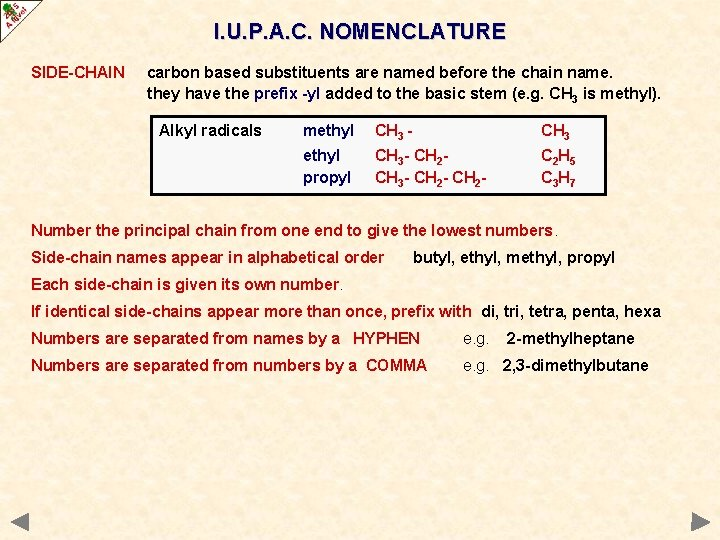I. U. P. A. C. NOMENCLATURE SIDE-CHAIN carbon based substituents are named before the chain name. they have the prefix -yl added to the basic stem (e. g. CH 3 is methyl). Alkyl radicals methyl CH 3 - CH 3 ethyl propyl CH 3 - CH 2 - C 2 H 5 C 3 H 7 Number the principal chain from one end to give the lowest numbers. Side-chain names appear in alphabetical order butyl, ethyl, methyl, propyl Each side-chain is given its own number. If identical side-chains appear more than once, prefix with di, tri, tetra, penta, hexa Numbers are separated from names by a HYPHEN e. g. 2 -methylheptane Numbers are separated from numbers by a COMMA e. g. 2, 3 -dimethylbutaneI. U. P. A. C. NOMENCLATURE SIDE-CHAIN carbon based substituents are named before the chain name. they have the prefix -yl added to the basic stem (e. g. CH 3 is methyl). Alkyl radicals methyl CH 3 - CH 3 ethyl propyl CH 3 - CH 2 - C 2 H 5 C 3 H 7 Number the principal chain from one end to give the lowest numbers. Side-chain names appear in alphabetical order butyl, ethyl, methyl, propyl Each side-chain is given its own number. If identical side-chains appear more than once, prefix with di, tri, tetra, penta, hexa Numbers are separated from names by a HYPHEN e. g. Numbers are separated from numbers by a COMMA e. g. 2, 3 -dimethylbutane Example longest chain 8 (it is an octane) 3, 4, 6 are the numbers NOT 3, 5, 6 order is ethyl, methyl, propyl 3 -ethyl-5 -methyl-4 -propyloctane 2 -methylheptane CH 3 CH 2 CH 3 CH 2 CH CH CH 2 CH 3I. U. P. A. C. NOMENCLATURE Apply the rules and name these alkanes THE ANSWERS ARE ON THE NEXT SLIDE CH 3 CH 2 CH 3 CH 2 CH CH 3 CH 2 CH CH CH 3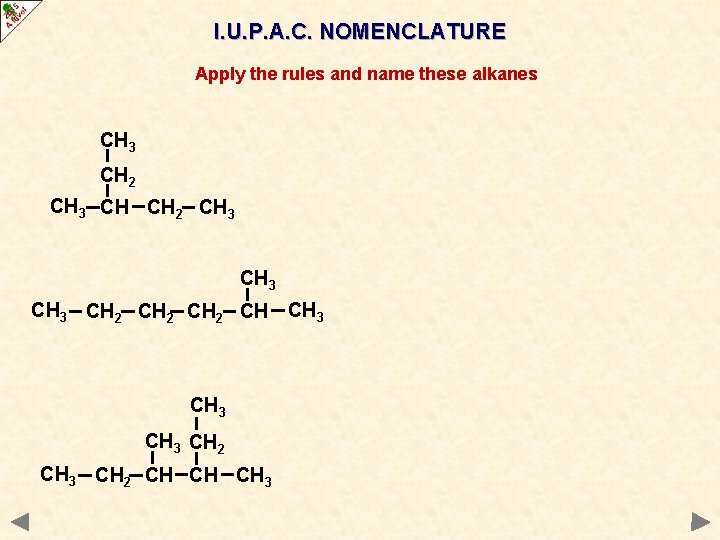I. U. P. A. C. NOMENCLATURE Apply the rules and name these alkanes CH 3 CH 2 CH 3 CH 2 CH CH 3 CH 2 CH CH CH 3I. U. P. A. C. NOMENCLATURE Apply the rules and name these alkanes Longest chain = 5 so it is a pentane CH 3 A CH 3, methyl, group is attached to the third carbon from one end. . . CH 2 CH 3 CH CH 2 CH 3 3 -methylpentane CH 3 CH 2 CH CH 3 CH 2 CH CH CH 3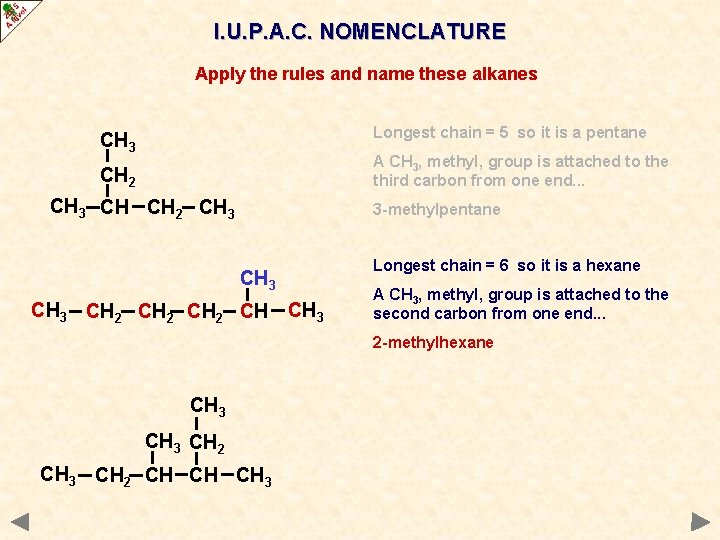I. U. P. A. C. NOMENCLATURE Apply the rules and name these alkanes Longest chain = 5 so it is a pentane CH 3 A CH 3, methyl, group is attached to the third carbon from one end. . . CH 2 CH 3 CH CH 2 CH 3 3 -methylpentane CH 3 CH 2 CH CH 3 Longest chain = 6 so it is a hexane A CH 3, methyl, group is attached to the second carbon from one end. . . 2 -methylhexane CH 3 CH 2 CH CH CH 3I. U. P. A. C. NOMENCLATURE Apply the rules and name these alkanes Longest chain = 5 so it is a pentane CH 3 A CH 3, methyl, group is attached to the third carbon from one end. . . CH 2 CH 3 CH CH 2 CH 3 3 -methylpentane CH 3 CH 2 CH CH 3 Longest chain = 6 so it is a hexane A CH 3, methyl, group is attached to the second carbon from one end. . . 2 -methylhexane CH 3 CH 2 CH CH CH 3 Longest chain = 6 so it is a hexane CH 3, methyl, groups are attached to the third and fourth carbon atoms (whichever end you count from). 3, 4 -dimethylhexaneNAMING ALKENES Length In alkenes the principal chain is not always the longest chain It must contain the double bond the name ends in -ENE Position Count from one end as with alkanes. Indicated by the lower numbered carbon atom on one end of the C=C bond 5 4 3 2 1 CH 3 CH 2 CH=CHCH 3 Side-chain is pent-2 -ene (NOT pent-3 -ene) Similar to alkanes position is based on the number allocated to the double bond 1 2 3 4 CH 2 = CH(CH 3)CH 2 CH 3 2 -methylbut-1 -ene 1 2 3 4 CH 2 = CHCH(CH 3)CH 3 3 -methylbut-1 -ene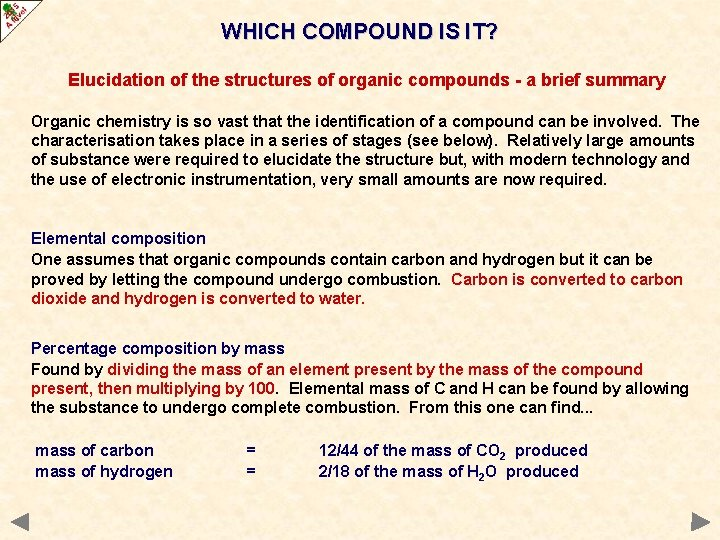WHICH COMPOUND IS IT? Elucidation of the structures of organic compounds - a brief summary Organic chemistry is so vast that the identification of a compound can be involved. The characterisation takes place in a series of stages (see below). Relatively large amounts of substance were required to elucidate the structure but, with modern technology and the use of electronic instrumentation, very small amounts are now required. Elemental composition One assumes that organic compounds contain carbon and hydrogen but it can be proved by letting the compound undergo combustion. Carbon is converted to carbon dioxide and hydrogen is converted to water. Percentage composition by mass Found by dividing the mass of an element present by the mass of the compound present, then multiplying by 100. Elemental mass of C and H can be found by allowing the substance to undergo complete combustion. From this one can find. . . mass of carbon mass of hydrogen = = 12/44 of the mass of CO 2 produced 2/18 of the mass of H 2 O producedINVESTIGATING MOLECULES Empirical formula The simplest ratio of elements present in the substance. It is calculated by dividing the mass or percentage mass of each element by its molar mass and finding the simplest ratio between the answers. Empirical formula is converted to the molecular formula using molecular mass.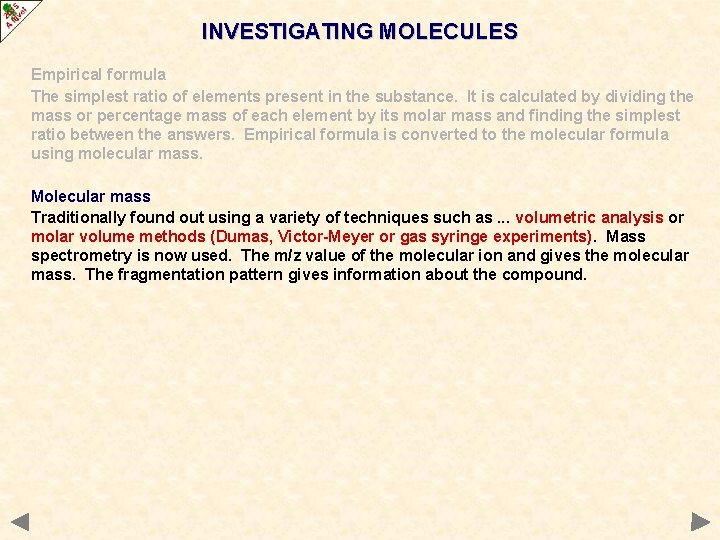INVESTIGATING MOLECULES Empirical formula The simplest ratio of elements present in the substance. It is calculated by dividing the mass or percentage mass of each element by its molar mass and finding the simplest ratio between the answers. Empirical formula is converted to the molecular formula using molecular mass. Molecular mass Traditionally found out using a variety of techniques such as. . . volumetric analysis or molar volume methods (Dumas, Victor-Meyer or gas syringe experiments). Mass spectrometry is now used. The m/z value of the molecular ion and gives the molecular mass. The fragmentation pattern gives information about the compound.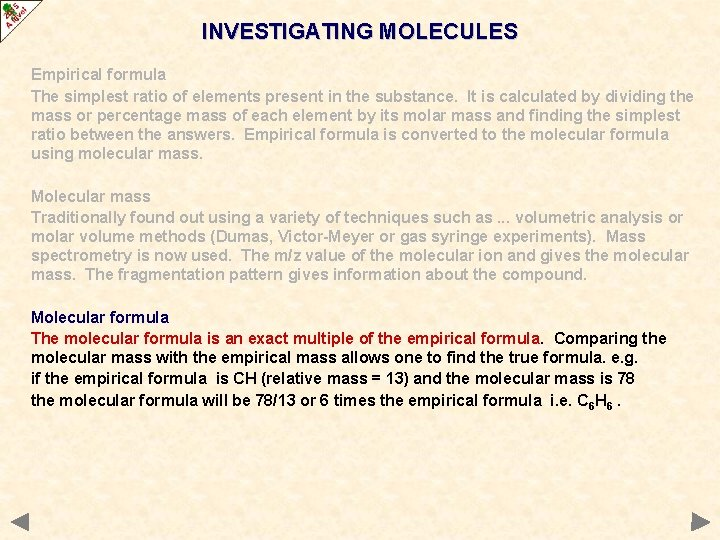INVESTIGATING MOLECULES Empirical formula The simplest ratio of elements present in the substance. It is calculated by dividing the mass or percentage mass of each element by its molar mass and finding the simplest ratio between the answers. Empirical formula is converted to the molecular formula using molecular mass. Molecular mass Traditionally found out using a variety of techniques such as. . . volumetric analysis or molar volume methods (Dumas, Victor-Meyer or gas syringe experiments). Mass spectrometry is now used. The m/z value of the molecular ion and gives the molecular mass. The fragmentation pattern gives information about the compound. Molecular formula The molecular formula is an exact multiple of the empirical formula. Comparing the molecular mass with the empirical mass allows one to find the true formula. e. g. if the empirical formula is CH (relative mass = 13) and the molecular mass is 78 the molecular formula will be 78/13 or 6 times the empirical formula i. e. C 6 H 6.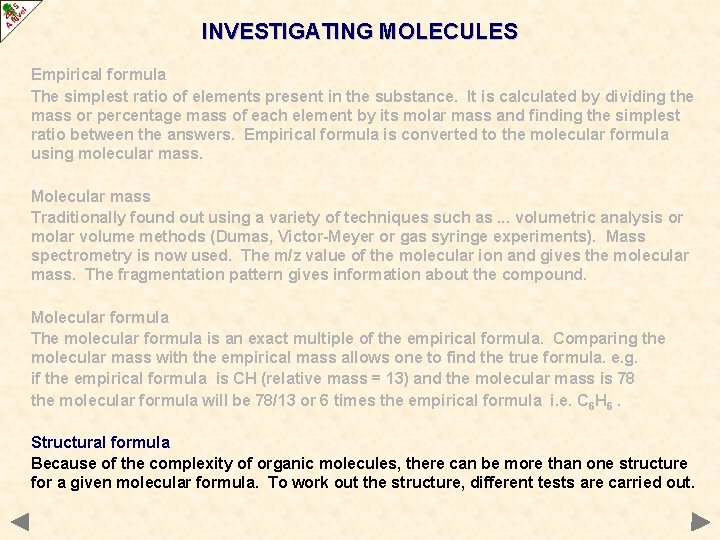INVESTIGATING MOLECULES Empirical formula The simplest ratio of elements present in the substance. It is calculated by dividing the mass or percentage mass of each element by its molar mass and finding the simplest ratio between the answers. Empirical formula is converted to the molecular formula using molecular mass. Molecular mass Traditionally found out using a variety of techniques such as. . . volumetric analysis or molar volume methods (Dumas, Victor-Meyer or gas syringe experiments). Mass spectrometry is now used. The m/z value of the molecular ion and gives the molecular mass. The fragmentation pattern gives information about the compound. Molecular formula The molecular formula is an exact multiple of the empirical formula. Comparing the molecular mass with the empirical mass allows one to find the true formula. e. g. if the empirical formula is CH (relative mass = 13) and the molecular mass is 78 the molecular formula will be 78/13 or 6 times the empirical formula i. e. C 6 H 6. Structural formula Because of the complexity of organic molecules, there can be more than one structure for a given molecular formula. To work out the structure, different tests are carried out.INVESTIGATING MOLECULES Empirical formula The simplest ratio of elements present in the substance. It is calculated by dividing the mass or percentage mass of each element by its molar mass and finding the simplest ratio between the answers. Empirical formula is converted to the molecular formula using molecular mass. Molecular mass Traditionally found out using a variety of techniques such as. . . volumetric analysis or molar volume methods (Dumas, Victor-Meyer or gas syringe experiments). Mass spectrometry is now used. The m/z value of the molecular ion and gives the molecular mass. The fragmentation pattern gives information about the compound. Molecular formula The molecular formula is an exact multiple of the empirical formula. Comparing the molecular mass with the empirical mass allows one to find the true formula. e. g. if the empirical formula is CH (relative mass = 13) and the molecular mass is 78 the molecular formula will be 78/13 or 6 times the empirical formula i. e. C 6 H 6. Structural formula Because of the complexity of organic molecules, there can be more than one structure for a given molecular formula. To work out the structure, different tests are carried out.INVESTIGATING MOLECULES Chemical reactions can identify the functional group(s) present. Spectroscopy IR detects bond types due to absorbance of i. r. radiation NMR gives information about the position and relative numbers of hydrogen atoms present in a molecule By comparison of IR or NMR spectra and mass spectrometry Confirmation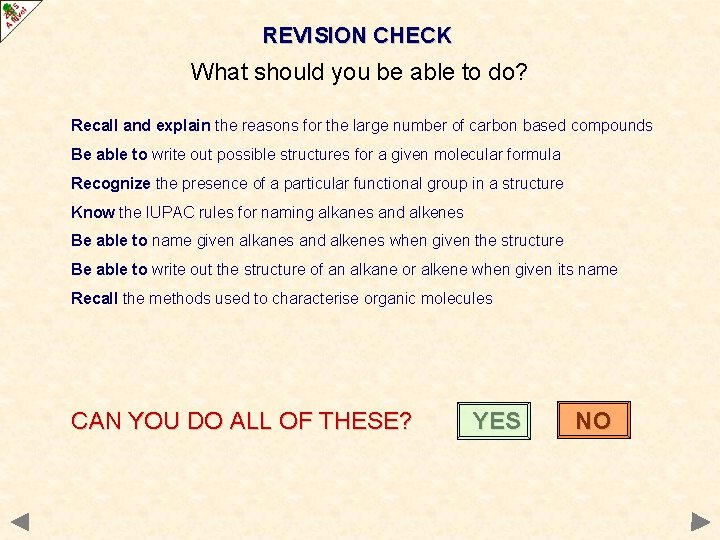REVISION CHECK What should you be able to do? Recall and explain the reasons for the large number of carbon based compounds Be able to write out possible structures for a given molecular formula Recognize the presence of a particular functional group in a structure Know the IUPAC rules for naming alkanes and alkenes Be able to name given alkanes and alkenes when given the structure Be able to write out the structure of an alkane or alkene when given its name Recall the methods used to characterise organic molecules CAN YOU DO ALL OF THESE? YES NOYou need to go over the relevant topic(s) again Click on the button to return to the menuWELL DONE! Try some past paper questions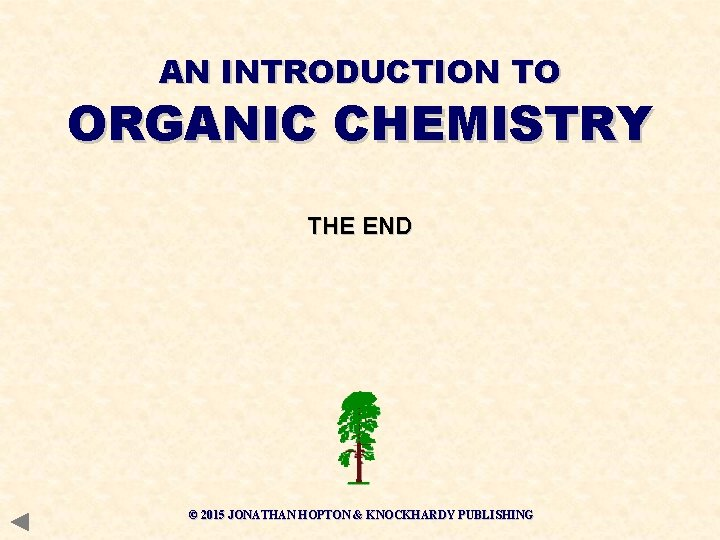AN INTRODUCTION TO ORGANIC CHEMISTRY THE END © 2015 JONATHAN HOPTON & KNOCKHARDY PUBLISHING